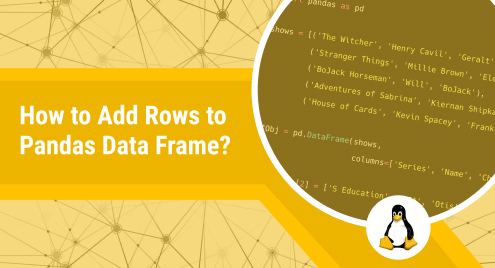# How to Add Rows to Pandas Data Frame?

Pandas is a powerful tool for manipulating and analyzing data, and one of the most common tasks you’ll need to do with Pandas is adding or inserting rows to a Data Frame. Whether working with a small dataset or a large one, adding rows to a Data Frame is an essential skill for any programmer/data analyst.

This Python post will explain all the possible methods to add rows to Pandas Data Frame.

## How to Add/Insert Rows into a Data Frame?

Multiple approaches are used in Python to add or insert a row to the Pandas DataFrame. The most frequently used approaches are the append() function, pandas.concat(), and the DataFrame.loc method. Let’s discuss each approach one by one.

### Approach #1: Add Rows Into a Data Frame Using the append()

The most popularly used function for inserting a row into a data frame is the append() function. It appends/inserts a row at the available space.

#### Code:

First, you need to import the Pandas library; then, create a dictionary and pass it to the pandas.DataFrame() to get a 2-D Pandas dataframe. After that, use the append() function to add a new row at the end of the given dataframe.

``````import pandas

inputVal = {'empName':['Paul', 'Vince', 'Amanda'],
'empAge':[34, 25, 35],
'empDesig':['CEO', 'HR', 'Project Manager']
}

newVal = {'empName':'Jordan', 'empAge':29, 'empDesig':'Designer'}
dataFrame = dataFrame.append(newVal, ignore_index=True)

print('\n Modified Dataframe: \n', dataFrame)
``````

#### Output:### Approach #2: Add Rows Into Data Frame Using DataFrame.loc

The “.loc” approach adds/appends a single row at the bottom of the selected DataFrame. You can use the len() function to determine the location/position at which you want to append a new row. The following coding example will demonstrate how to add/append a row at the end of a DataFrame.

#### Code:

First, you must import the Pandas library; then, create a dictionary and pass it to the pandas.DataFrame() to get a 2-D Pandas dataframe. Finally, use the dataframe.loc() method to append a new row at the bottom/end of the selected dataframe.

``````import pandas

inputVal = {'empName':['Paul', 'Vince', 'Amanda'],
'empAge':[34, 25, 35],
'empDesig':['CEO', 'HR', 'Project Manager']
}

dataFrame = pandas.DataFrame(inputVal)
print('Original Dataframe: \n', dataFrame)

dataFrame.loc[len(dataFrame.index)] = ['Jordan', 29, 'Programmer']
print('\n Modified Dataframe: \n', dataFrame)
``````

#### Output:### Approach #3: Add Rows Into Data Frame Using pandas.concat()

In Python, the “pandas.concat()” function takes two dataframes and returns a single combined or concatenated dataframe. If you want to insert a new row into a dataframe using the concat() function, you must insert the new row into a new dataframe and then concatenate it with the given dataframe.

#### Code:

First, you must import the Pandas library; then, create two dictionaries and use the pandas.DataFrame() to get 2-D Pandas data frames. Finally, use the concat() function to add a new row at the end of the given dataframe.

``````import pandas

inputVal = {'empName':['Paul', 'Vince', 'Amanda'],
'empAge':[34, 25, 35],
'empDesig':['CEO', 'HR', 'Project Manager']
}

dataFrame = pandas.DataFrame(inputVal)
print('Original Dataframe: \n', dataFrame)

inputVal_1 = {'empName':['Jordan'], 'empAge':, 'empDesig':['Designer']}
dataFrame_1 = pandas.DataFrame(inputVal_1)

newDataFrame = pandas.concat([dataFrame, dataFrame_1], ignore_index = True)
print('\n Modified Dataframe: \n', newDataFrame)
``````

#### Output:## Conclusion

In Python, the append() function, pandas.concat() function, and the DataFrame.loc method is used to add or insert a new row into a data frame. The pandas.DataFrame() is used to get a 2-D Pandas data frame. After that, the append() and DataFrame.loc methods are used to append or insert a new row to the given data frame, while the concat() function is used to concatenate/combine two data frames. This tutorial explained using different functions to insert or add a new row into a data frame.

Visit Linux Genie for more Python tutorials.